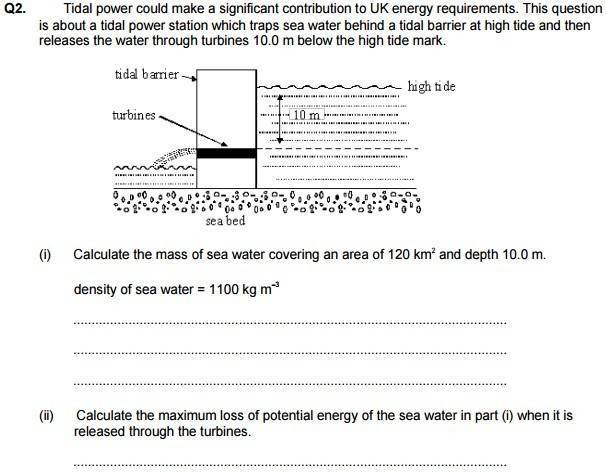# Calculating the loss of Potential Energy of water in turbine

TiernanW

## Homework Statement## Homework Equations

Power = Work Done / Time
Work Done = Force * Displacement
PE = mass * gravity * height

## The Attempt at a Solution

I got the first part (i) right, so the mass of the sea water is 1.32 x 10^12 kg.

Part (ii) is the bit I am stuck on. My thoughts were that it must fall 10m, so the max loss in PE is (1.32 x 10^12)(9.81)(10), but the mark scheme says the height is 5m, so that it is (1.32 x 10^12)(9.81)(5). Why do we take the height to be 5m here when it falls through 10m?

Mycelium
I could be wrong, but if we use the 10m as a scale, it looks like there is a distance of less than 5m from the turbines to the sea bed. The water is being released from the turbines so the gpe lost should be 5mg as a maximum value. It shouldn't be 10, at least, because it isn't being released from that height.

TiernanW
I could be wrong, but if we use the 10m as a scale, it looks like there is a distance of less than 5m from the turbines to the sea bed. The water is being released from the turbines so the gpe lost should be 5mg as a maximum value. It shouldn't be 10, at least, because it isn't being released from that height.
Ah I can see where you are coming from. The loss of potential for the water going INTO the turbines would be 10, but for the water leaving it is less than that. We aren't given the total height though. That is odd.

Post #2 isn't right. The distance from the turbine exit to the sea bed is irrelevant.

What happens to the water level as the reservoir empties?
In other words, is all the water 10m above the exit? Or just an infinitesimal slice?

TiernanW
Post #2 isn't right. The distance from the turbine exit to the sea bed is irrelevant.

What happens to the water level as the reservoir empties?
In other words, is all the water 10m above the exit? Or just an infinitesimal slice?
This I had also thought about, but I didn't really know how to approach it because the equation looks for a value of h. The water level falls. Is it like the average water level because 5 is half-way between 10 and 0?

•CWatters
This I had also thought about, but I didn't really know how to approach it because the equation looks for a value of h. The water level falls. Is it like the average water level because 5 is half-way between 10 and 0?
Yes, it's the height of the centre of mass of the water above the turbine.
It happens to be half way as the planar cross section of the water is constant, if the reservoir walls were sloped( like most real hydro dam reservoirs are) then it wouldn't be half way.

•CWatters
late347
why is the centre of gravity the crucial fact here?

I suppose one could say that when the center of gravity has "shifted downwards" the amount of 10m. Then the entire watervolume above the turbine would have fallen below the waterline at the turbine level... In this case it seems the watervolume in question, from the oceanside, would have gone through the turbine to the beachside of the wall. (?)

wouldn't each "segment of water" have different potential energy because each height is different relative to the fixed location of the turbine at the base of the wall.

If you had very short height cuboids (of water) then each cuboid (area * height)would have different energy. because each cuboud has differing altitude compared to the other ones.

http://www.engineeringtoolbox.com/hydropower-d_1359.html

•